# Converting Word Vectors Into Document Vectors Using the Gensim Library

0
111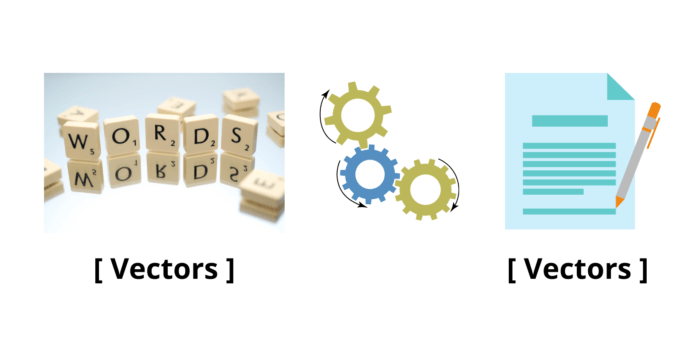In Natural Language Processing (NLP), word embedding refers to the process of representing words into numerical format or vectors so that the machines can understand the text data and perform the required operations on it. The most common method for converting words from text data into vectors is using the Word2Vec algorithm. It uses a simple neural network model to learn word associations from a text corpus. Once trained, it can find synonymous words for given words, find similarities between two or more words, and perform many more tasks. Moreover, it converts all the words in the data into their separate unique vectors during this process.

The Word2Vec algorithm consists of two model architectures – continuous bag of words (CBOW) and skip-grams. The model predicts the current word from a window of surrounding context words in the CBOW architecture. The sequence of the words in the context does not affect the outcome. The model predicts the surrounding window of context words in the continuous skip-gram architecture based on the current word.

A more sophisticated algorithm is also present, which, unlike word2vec, can convert sentences or entire documents into vectors at once. This algorithm is known as the Doc2Vec algorithm. Doc2Vec algorithm also has two model architectures – distributed memory version of paragraph vectors (PV-DM) and distributed bag of words version of paragraph vectors (PV-DBOW).  Both these algorithms, Word2Vec and Doc2Vec, can be conveniently implemented using the Gensim library

Although both these algorithms are highly efficient and easy to implement, the model may take a considerable amount of time for training with larger datasets. Hence, to reduce time and the computational load on the system, we can develop an algorithm that can construct the document vectors by taking word vectors for a particular document. This article demonstrates a similar algorithm that takes the word vectors as input and generates the document vectors for the given data.

## Structure of The Algorithm to Convert Word Vectors Into Document Vectors

The code accepts word vectors of all the words in the text data and generates document vectors or sentence vectors based on the words present in each sentence. It adds all the word vectors of the words present in a particular sentence and then normalizes the resulting vector by dividing it by the total number of words present in that sentence. In other words, it averages the word vectors of all the words present in a particular sentence to generate the document vector for that sentence. For instance, suppose the word vectors for the words ‘That’, ‘car’, ‘is’ and ‘nice’ are [1 0 1 1], [0 1 1 1], [1 0 0 1] and [1 1 1 1] respectively. Then, the code will produce a document vector [0.75 0.5 0.75 1]  for the sentence “That car is nice.”.

## Implementation of The Algorithm in Python Programming Language

Before we implement the algorithm, we need to extract the word vectors for the text data as the algorithm accepts word vectors for generating the document vectors. For converting the text data into word vectors, we use the word2vec model from the Gensim library.

### Importing the Required Libraries and The Text Data

This tutorial requires importing a few external libraries. These include the Numpy, Pandas, Gensim, and the Nltk library. The uses of these libraries are specified below.

1. The Numpy library for performing operations on arrays as the word and doc vectors are in the form of arrays.
2. The Pandas library for converting the text into a data frame.
3. The Nltk library for preprocessing the text data.
4. The Gensim library for implementing the word2vec model to obtain the word vectors of the text data.

##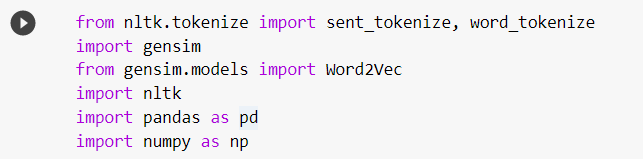For this tutorial, we will use a text paragraph copied from Wikipedia. This text is stored in a variable named txt.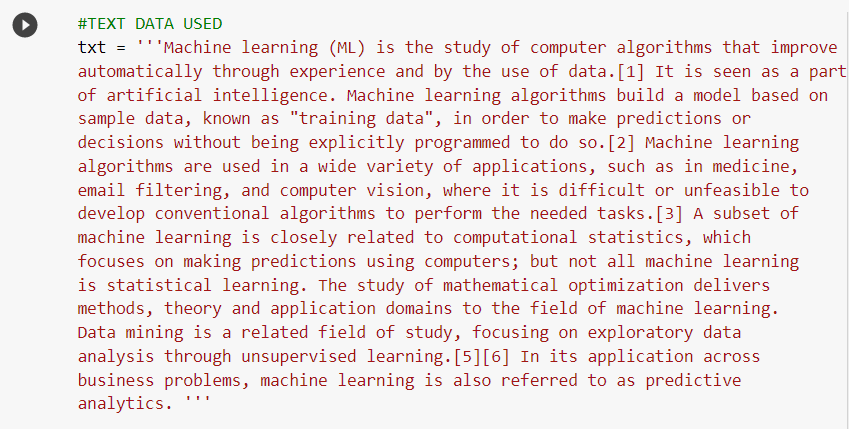Preprocessing The Text Data

Before implementing the word2vec algorithm, the data must be preprocessed or cleaned. Preprocessing includes removal of the stopwords, lowering all the words, removal of punctuations and special symbols, lemmatization, tokenization, etc.

First, the data is tokenized into different sentences with the help of the sent_tokenize() tokenizer from the Nltk library.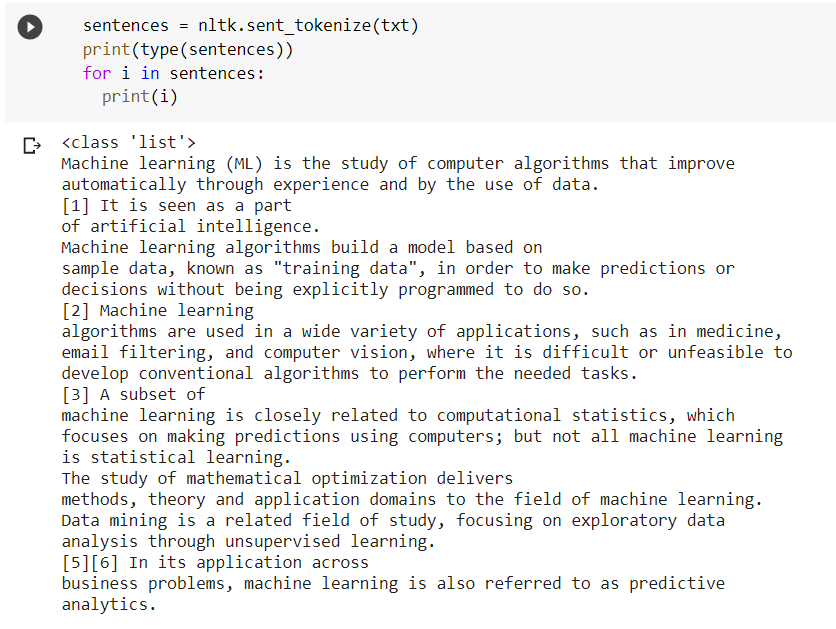These sentences are stored in a proper data frame using the Pandas library.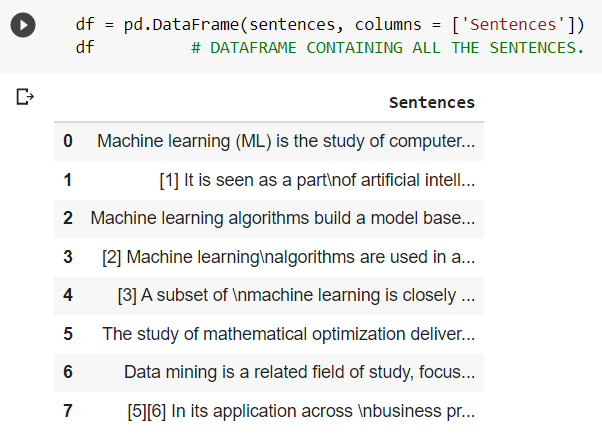As observed, the data contains eight sentences. The Gensim library provides a package named simple_preprocess that makes cleaning the data very convenient.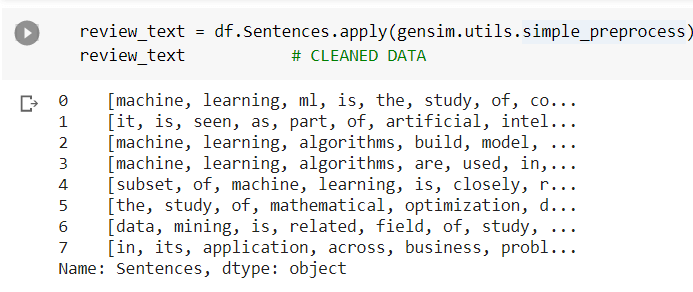The data has been cleaned and is ready to be fed to the model.

### The word2vec model can be built, trained, and saved as shown in the following code snippets.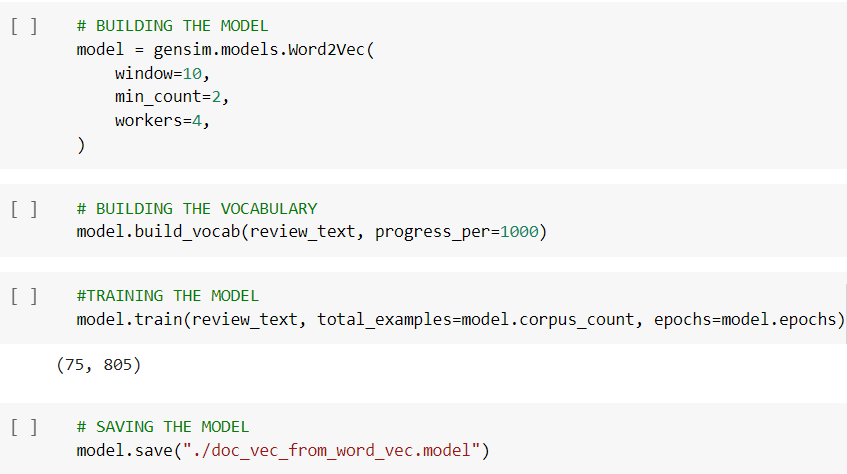The model has been built and trained. We save the word vectors of all the words in a dictionary (word_vec) for further use.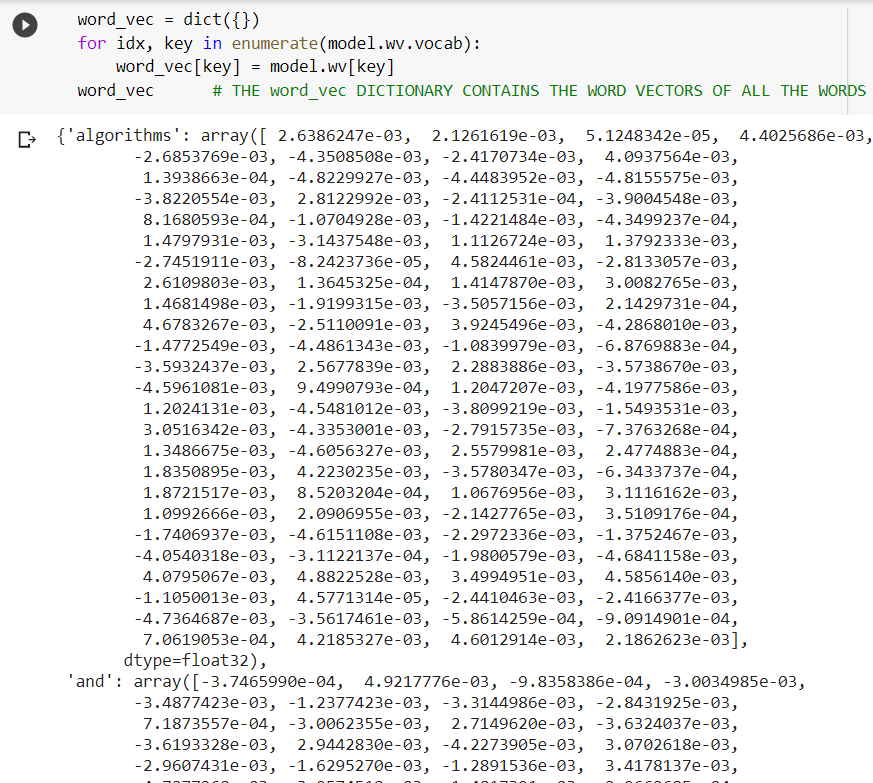Actual Implementation of The Algorithm to Convert Word Vectors Into Document Vectors

The following code snippet demonstrates the code for converting word vectors into document vectors.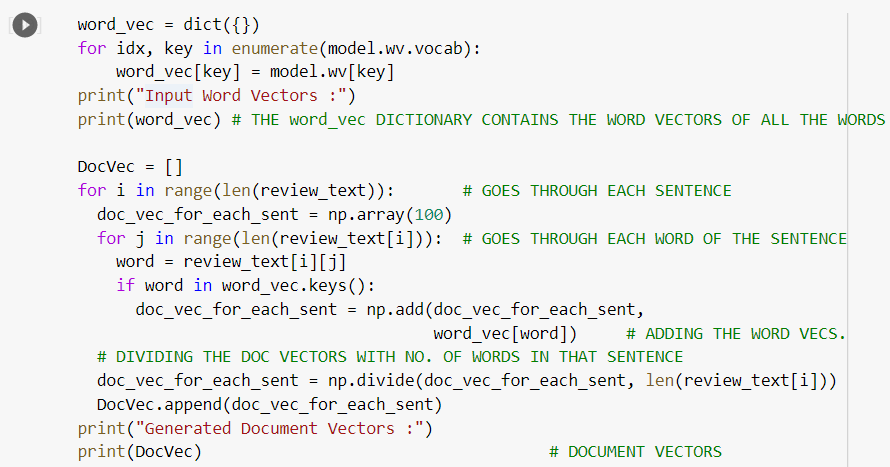The code uses two nested for-loops – the outer loop iterates through each sentence present in the data frame (review_text), and the inner loop iterates through each word of the particular sentence. An empty list named DocVec is created for storing the document vectors. Also, another empty array named doc_vec_for_each_sent is created for temporarily storing the document vectors of particular sentences.  Inside the inner for-loop, the words present in a sentence are temporarily stored in a variable named word. If the word vector associated with this word is present in the dictionary (word_vec), which contains all the word vectors, that word vector is added to the empty array. This loop continues till the word vectors associated with all the words present in that sentence are added to the array (doc_vec_for_each_sent). Once this step is completed, the array is divided by the number of words present in that sentence to normalize the values of the document vector. Then the final document vector generated for that particular sentence is appended to the list DocVec. This process repeats for all the sentences in the text data producing a vector that contains the sentence vectors associated with all the sentences present in the data. Snippets from the output of the code are shown below.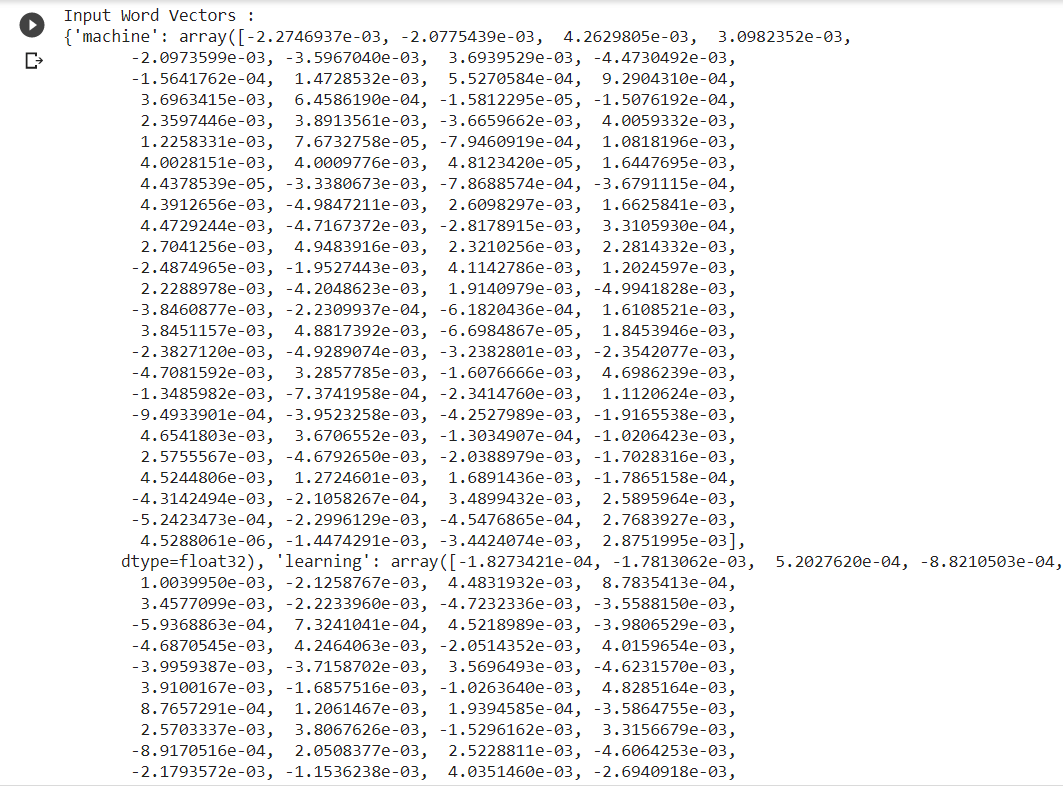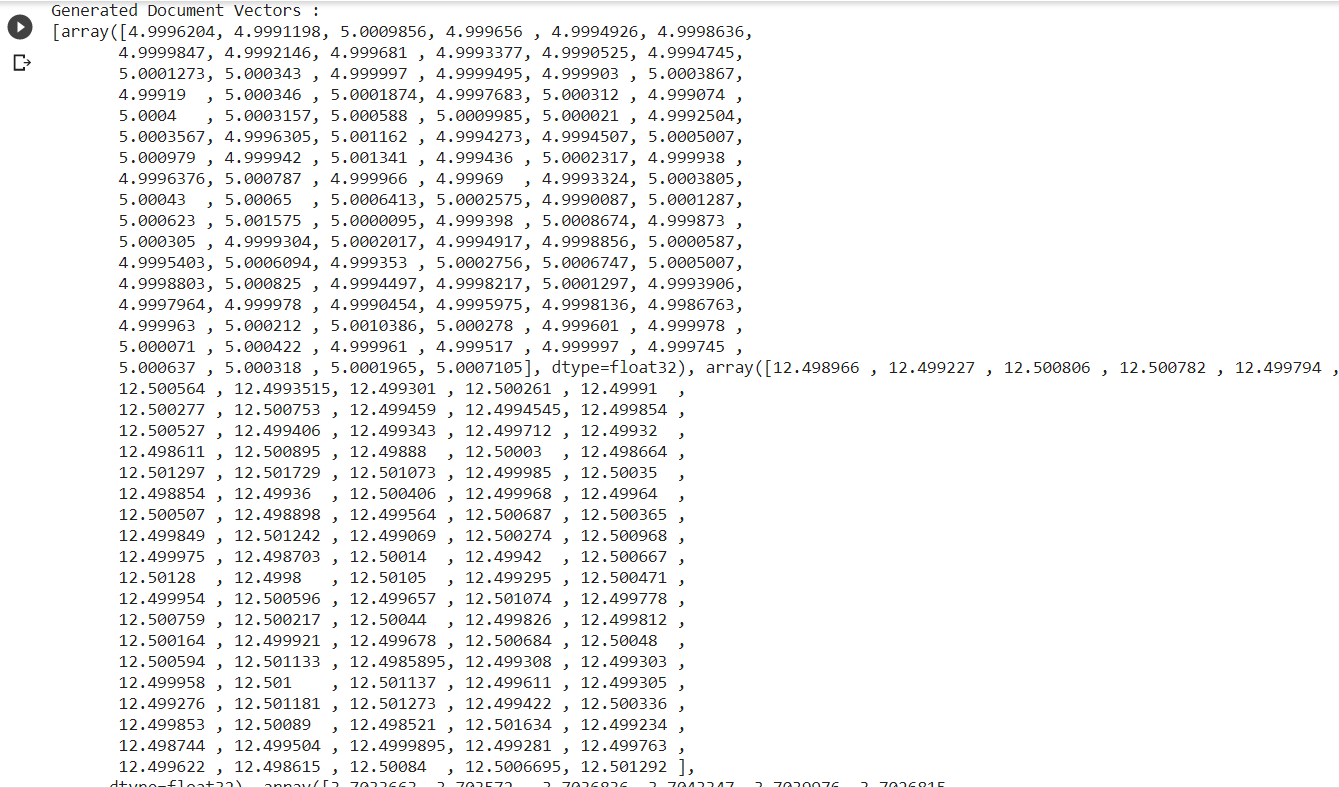This logic makes it extremely easy to generate document vectors from already available word vectors hence saving the time required to train the models. This logic can also be implemented in numerous other ways or some other algorithm can also be developed for generating document vectors directly from word vectors to save time and lessen the computational load.

Also Read: Text Preprocessing In Natural Language Processing